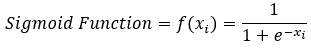# Machine Learning and Credit Risk (part 3) – Multinomial Logistic Regression

Logistic regression has been a reliable tool in many Statisticians/Economists toolkit for many years when dealing with binary problems where the output is 0/1, True/False, or any variation of a dichotomous problem. But the reality is that Multinomial Logistic regression is a very important ‘algorithm’ in the machine learning sphere.

Multinomial logistic regression is an extension of the binary logistic regression which allows for more than two categories of the dependent or outcome variable. While Logistic regression is commonly used for discrete binary problems, Multinomial Logistic regression is built with an eye towards multi-class classification or regression problems.

A Logistic classifier uses either a Sigmoid or Softmax function (both are variations of the commonly known Logistic function):

• Sigmoid function:  binary classification or regression using logistic regression model.• Softmax function: multi-classification or multinomial regression using multinomial logistic regression model.Multinomial Logistic regression models are ideal for forecasting credit migration matrices.  The model can use effect of independent variables and predict the probabilities of different possible outcomes.

• Logistic Regressions do not perform well when feature space is too large
• Doesn’t handle large number of categorical features/variables well
• Relies on transformations for non-linear features

Figure 5 displays the conditional matrices derived by the Multinomial Logistic Regression approach. It displays the forecasted migration matrices conditioned on Baseline, Adverse, and Severely Adverse economic conditions.```library("RTransProb")

for (i in c(24, 25, 26)) {
data &lt;- data

attach(data)
data2 &lt;- data[order(ID, Date),]
detach(data)

data &lt;- data2
rm(data2)

histData &lt;- histData

predData_mnl2 &lt;- predData_mnl_Baseline
predData_mnl2 &lt;span 				data-mce-type="bookmark" 				id="mce_SELREST_start" 				data-mce-style="overflow:hidden;line-height:0" 				style="overflow:hidden;line-height:0" 			&gt;&lt;/span&gt;&lt;- subset(
predData_mnl2,
X == i,
select = c(Market.Volatility.Index..Level.,
D_B, D_C, D_D, D_E, D_F, D_G
)
)

indVars   = c("Market.Volatility.Index..Level."
)

startDate = "1991-08-16"
endDate   = "2007-08-16"
method    = "cohort"
snapshots = 1
interval  = 1
ref       = 'A'
depVar    = c("end_rating")
ratingCat = c("A", "B", "C", "D", "E", "F", "G", "N")
defind    = "N"
wgt       = "mCount"

transForecast_mnl_out &lt;-
transForecast_mnl(
data, histData, predData_mnl2, startDate, endDate, method,
interval, snapshots, defind, ref, depVar, indVars, ratingCat, wgt
)
output &lt;- transForecast_mnl_out\$mnl_Predict
print(output)

}

```